Next: Solving for the calibration Up: Computer Vision IT412 Previous: Lecture 9

Subsections

# Camera calibration

We will now return to image formation and camera geometry in a bit more detail to determine how one calibrates a camera to determine the relationship between what appears on the image (or retinal) plane and where it is located in the 3D world.

Imagine we have a three dimensional coordinate system whose origin is at the centre of projection and whose Z axis is along the optical axis, as shown in figure 1. This coordinate system is called the standard coordinate system of the camera. A point M on an object with coordinates (X,Y,Z) will be imaged at some point m = (x, y) in the image plane. These coordinates are with respect to a coordinate system whose origin is at the intersection of the optical axis and the image plane, and whose x and y axes are parallel to the X and Y axes. The relationship between the two coordinate systems (c,x,y) and (C,X,Y,Z) is given by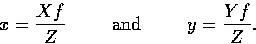(1)
This can be written linearly in homogeneous coordinates as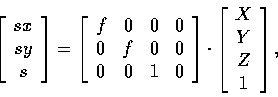where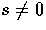is a scale factor.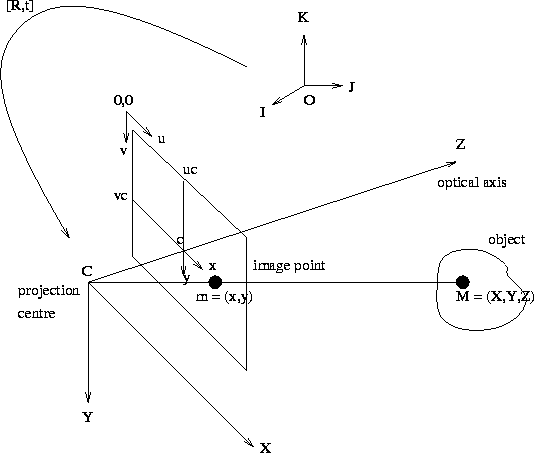Now, the actual pixel coordinates (u,v) are defined with respect to an origin in the top left hand corner of the image plane, and will satisfy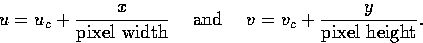(2)

We can express the transformation from three dimensional world coordinates to image pixel coordinates using a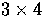matrix. This is done by substituting equation (1) into equation (2) and multiplying through by Z to obtain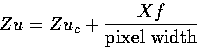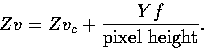In other words,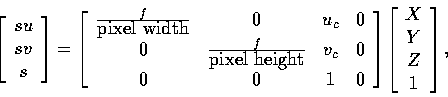where the scaling factor s has value Z. In short hand notation, we write this as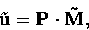where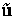represents the homogeneous vector of image pixel coordinates, P is the perspective projection matrix, and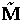is the homogeneous vector of world coordinates. Thus, a camera can be considered as a system that performs a linear projective transformation from the projective space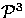into the projective plane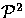.

There are five camera parameters, namely the focal length f, the pixel width, the pixel height, the parameter uc which is the u pixel coordinate at the optical centre, and the parameter vc which is the v pixel coordinate at the optical centre. However, only four separable parameters can be solved for as there is an arbitrary scale factor involved in f and in the pixel size. Thus we can only solve for the ratios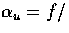pixel width and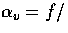pixel height. The parameters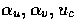and vc do not depend on the position and orientation of the camera in space, and are thus called the intrinsic parameters.

In general, the three dimensional world coordinates of a point will not be specified in a frame whose origin is at the centre of projection and whose Z axis lies along the optical axis. Some other, more convenient frame, will more likely be specified, and then we have to include a change of coordinates from this other frame to the standard coordinate system. Thus we have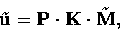where K is a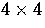homogeneous transformation matrix: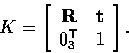The top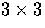corner is a rotation matrix R and encodes the camera orientation with respect to a given world frame; the final column is a homogeneous vector t capturing the camera displacement from the world frame origin. The matrix K has six degrees of freedom, three for the orientation, and three for the translation of the camera. These parameters are known as the extrinsic camera parameters.

Thecamera matrix P and thehomogeneous transform K combine to form a singlematrix C, called the camera calibration matrix. We can write the general form of C as a function of the intrinsic and extrinsic parameters: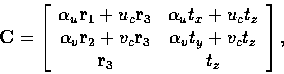(3)
where the vectors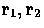, and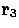are the row vectors of the matrix R, and t = (tx, ty, tz). The matrix C, like the matrix P, has rank three.

## Orthographic projection

Consider a translation of -f along the Z axis of the standard coordinate frame, so that the focal plane and the image plane are now coincident. Since there is no rotation involved in this transformation, it is easy to see that the camera calibration matrix is just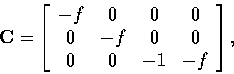where we are assuming that the pixel width and height are both 1. Now since C is defined up to a scale factor, this is the same as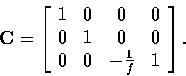Now, if we let f go to infinity, the matrix becomes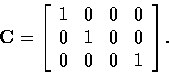This defines the transformation u = X and v = Y and is known as an orthographic projection parallel to the Z axis. It appears as the limit of the general perspective projection as the focal length f becomes large with respect to the distance Z of the camera from the object.Next: Solving for the calibration Up: Computer Vision IT412 Previous: Lecture 9
Robyn Owens
10/29/1997